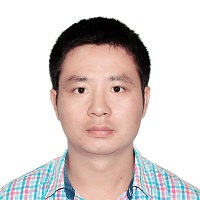Hessian Recovery Based Finite Element Methods for the Cahn-Hilliard Equation
Speaker
Dr. Hai-Long Guo
University of Melbourne
Abstract

In this talk, we talk about some new recovery based finite element methods for the 2D Cahn-Hilliard equation. One distinguishing feature of those methods is that we discretize the fourth-order differential operator in a standard \$C^0\$ linear finite elements space. Precisely, we first transform the fourth-order Cahn-Hilliard equation to its variational formulation in which only first-order and second-order derivatives are involved and then we compute the first and second-order derivatives of a linear finite element function by a least-squares fitting recovery procedure. When the underlying mesh is uniform meshes of regular pattern, our recovery scheme for the Laplacian operator coincides with the well- known five-point stencil. Another feature of the methods is some special treatments on Neumann type boundary conditions for reducing computational cost. The optimal-order convergence and energy stability are numerically proved through a series of benchmark tests. The proposed method can be regarded as a combination of the finite difference scheme and the finite element scheme.